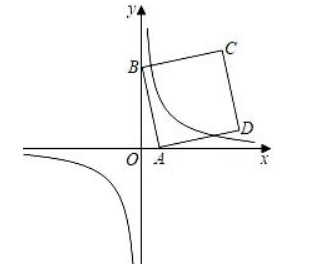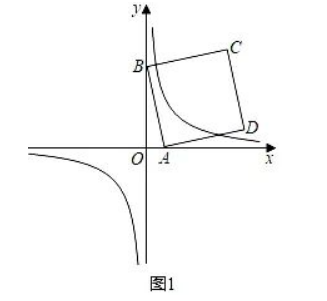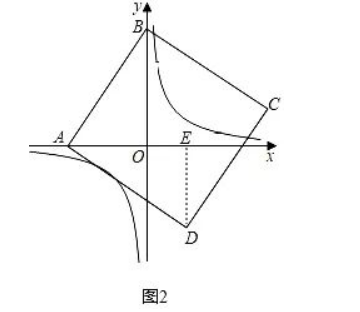(1) 已知直线 $y=k x-2$ 和扡物线 $y=x^2-2 x+3$ ，
① 当 $k=4$ 时, 求直线与抛物线的交点坐标;
②当 $k$ 为何值时, 直线与拋物线只有一个交点?

(2) 已知点 $A(a, 0)$ 是 $x$ 轴上的动点, $B(0,4 \sqrt{2})$, 以 $A B$ 为边在 $A B$ 右侧做正方形 $A B C D$, 当正方形 $2 \sqrt{2}$
$A B C D$ 的边与反比例函数 $y=\overline{\mathrm{x}}$ 的图象有 4 个交点时, 试求 $a$ 的取值范围.②联立两个函数并整理得: $x^2-(k+2) x+5=0$ ，
$\Delta=(-k-2)^2-4 \times 5=0$,

(2) ①当 $a>0$ 时, 如图1,$\triangle=(-4 a)^2-16 \times 2 a=0$, 解得: $a=2$,

②当 $a < 0$ 时, 如图2,(I) 当边 $A D$ 与双曲线有一个交点时,

\begin{aligned} & \because \angle B A O+\angle D A E=90^{\circ}, \angle D A E+\angle A D E=90^{\circ}, \\ & \therefore \angle A D E=\angle B A O, \\ & \because A B=A D, \angle A O B=\angle D E A=90^{\circ}, \\ & \therefore \triangle A O B \cong \triangle D E A(A A S), \\ & \therefore E D=A O=-a, A E=O B=4 \sqrt{2}, \\ & \text { 故点 } D(a+4 \sqrt{2}, a), \end{aligned}

$\Delta=\left(-a^2\right)^2-4 a \times(16)=0$ ，解得： $a=-4$ （不合题意值已舍去）;
(II) 当边 $B C$ 与双曲线有一个交点时，